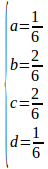# A partial derivation of the RK4 ODE-solver // RK4积分器的部分推导

RK4
is an ODE solver similar to euler’s method, with the iterator taking
the
form: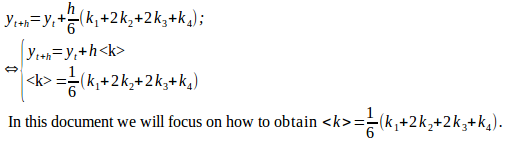RK-family
method relies on finding k_n iteratively, in RK4 the iteration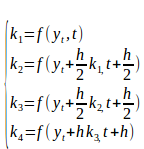However,
these relations
are
only useful

to derive k2, not from k1 directly, but
proxyed through
a complicated y. We now seek to eliminate this proxy. We wonder what
happened to the coefficients of k1 to k4. Thus, we should
taylor-expand k1 to k4, exploiting the aforementioned preserved
relation. Note this expansion has a 2D form if partial derivative is
taken. However we can exploit the principle of total derivative to
simplify the notation.(This is essentially dropping the dependency of
“f” on “y” to achieve a simpler notation).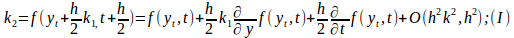By
the principle of total derivative: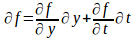Take
derivative wrt t: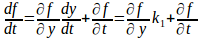Exchange
LHS with RHS, time both
sides
by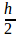gives: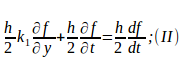substitue
( II ) into ( I ) gives: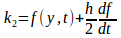Note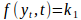,in
other words: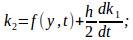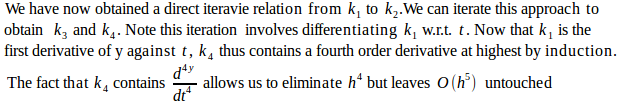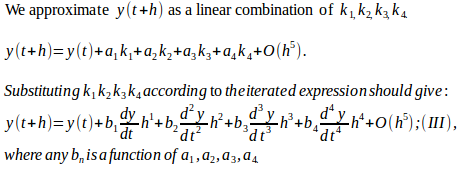Compare
(III) against well-known Taylor expansion of y( t+h ):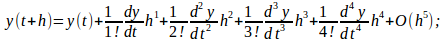We
obtain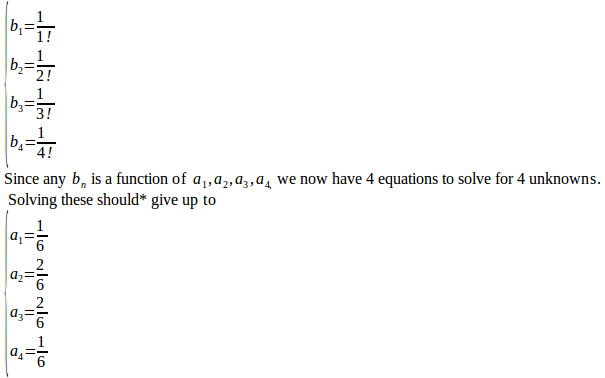*According
to Wikipedia, the full expansion of  (III) looks likeGiving
up to:and
thus